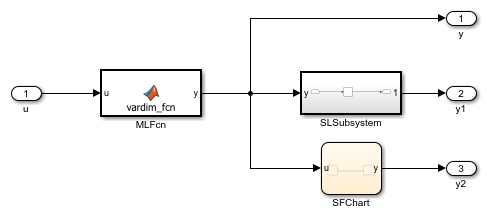# Generate Structured Text Code for Variable-Size Signals

Deploy applications such as machine learning models to your target PLC by generating code for variable-size signals. Verify the generated structured text code by generating test bench code and importing the generated test bench code into your target IDE.

### Model Description

The model consists of a simple MATLAB® Function Block, Stateflow® chart and a Simulink® subsystem. The MATLAB® Function Block generates a variable one-dimensional array based on whether the input signal `u` is greater than or less than zero. The Stateflow® chart has two states that transition between assigning the output variable `y` to the input variable `u` and multiplying `y` by two. The Simulink® subsystem has a `Sum of Elements` block that sums all the elements in `y`.

### Simulate and Generate Structured Text Code

```mdl = 'vardim_ex'; open_system(mdl) ```To generate structured text code for the `Subsystem` block, use the `plcgeneratecode` command.

```plcgeneratecode([mdl '/Subsystem']); ```

Close the model

```close_system(mdl) ```